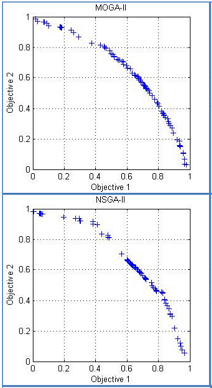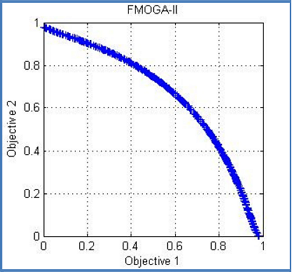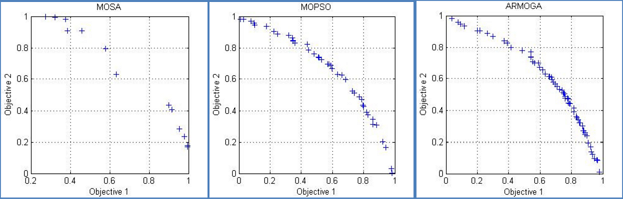# Comparison between Multiobjective Population-Based Algorithms in Mechanical Problem

Author(s):
H.E. Radhi, S.M. Barrans (University of Huddersfield)

Unlike single criterion optimization, in multi-objective problems it is not possible to have a single solution that optimizes all objectives, there usually exists a set of non-dominated solutions or Pareto optimal solutions. The first aim of this study was to perform a complete comprehensive comparison between multi-objective optimization methods by using the commercial modeFRONTIER software to solve the standard test problem SCH and FON and examining the efficiency of each method.

Six multi-objective population-based optimization algorithms were introduced for comparison of the effectiveness of each. The six algorithms examined in this study were: Multi-objective Genetic Algorithm (MOGA-II),Adaptive range Multi-objective Genetic Algorithm (ARMOGA), Fast Multi-objective Genetic Algorithm (FMOGA-II),Non-dominated Sorting Genetic Algorithm (NSGA-II), Multi-objective Particle Swarm Optimization (MOPSO) and Multi-objective Simulated Annealing (MOSA).Two numerical performance metrics and one visual criterion were chosen for qualitative and quantitative comparison : (1) Spacing metric (S) which indicates the distribution of the Pareto front in the objective space, and (2) the ratio between the number of resulting Pareto front members and the total number of fitness function calculations, and lastly (3) graphical representation of the Pareto fronts for discussion. These metrics were chosen to represent the quality, as well as speed of the algorithms by ensuring well distributed solution. To evaluate the performance of the algorithms two well-known objectives benchmark problems (SCH) and (FON), two quality indicators and one visual criterion have been used. According to experiments done with test functions and the parameter settings used, it can be conclude that:

• In convex problem (SCH) the FMOGA-II significantly outperforms 5 other algorithms in terms of hit rate and spacing metric.
• In concave problem (FON) the performance of FMOGA-II is still very well in both metrics and has a well-distributed solution over the Pareto front.

On the whole, the FMOGA-II outperforms all other types of algorithms and is suitable for use in convex concave multi-objective optimization problems.